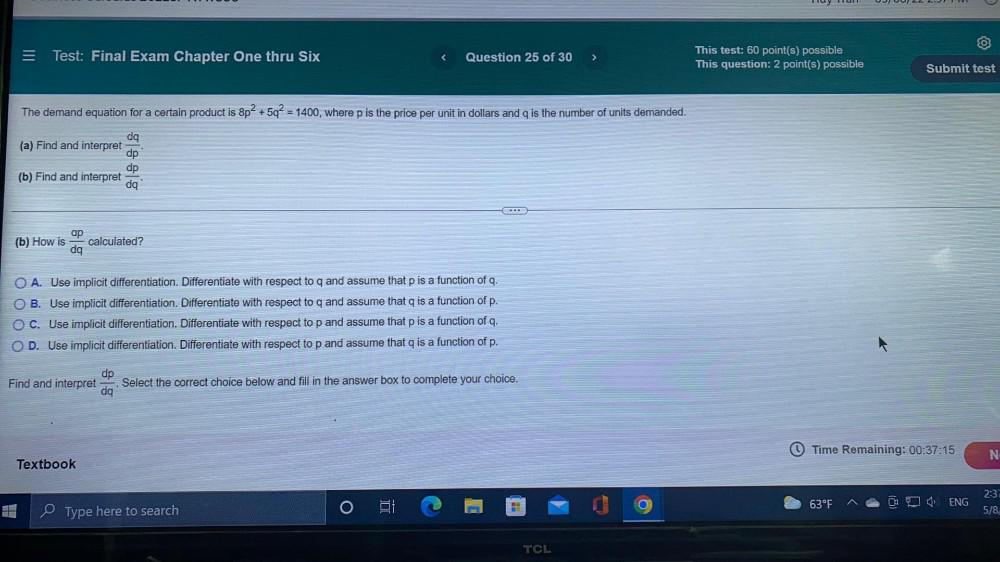Question:

# The demand equation for a certain product is 8p² +5q² = 1400, where p is the price per unit in dollars and q is the number of units demanded. (a) Find and interpret dq/dp. (b) Find and interpret dp/dThe demand equation for a certain product is 8p² +5q² = 1400, where p is the price per unit in dollars and q is the number of units demanded. (a) Find and interpret dq/dp. (b) Find and interpret dp/dq. (b) How is ap/dq calculated? ○ A. Use implicit differentiation. Differentiate with respect to q and assume that p is a function of q. ○ B. Use implicit differentiation. Differentiate with respect to q and assume that q is a function of p. ○ C. Use implicit differentiation. Differentiate with respect to p and assume that p is a function of q. ○ D. Use implicit differentiation. Differentiate with respect to p and assume that q is a function of p. Find and interpret dq/dp, Select the correct choice below and fill in the answer box to complete your choice.